# The GLIMMIX Procedure

### Example 45.15 Comparing Multiple B-Splines

This example uses simulated data to demonstrate the use of the nonpositional syntax (see the section Positional and Nonpositional Syntax for Contrast Coefficients for details) in combination with the experimental EFFECT statement to produce interesting predictions and comparisons in models containing fixed spline effects. Consider the data in the following DATA step. Each of the 100 observations for the continuous response variable `y` is associated with one of two groups.

```data spline;
input group y @@;
x = _n_;
datalines;
1    -.020 1    0.199 2    -1.36 1    -.026
2    -.397 1    0.065 2    -.861 1    0.251
1    0.253 2    -.460 2    0.195 2    -.108
1    0.379 1    0.971 1    0.712 2    0.811
2    0.574 2    0.755 1    0.316 2    0.961
2    1.088 2    0.607 2    0.959 1    0.653
1    0.629 2    1.237 2    0.734 2    0.299
2    1.002 2    1.201 1    1.520 1    1.105
1    1.329 1    1.580 2    1.098 1    1.613
2    1.052 2    1.108 2    1.257 2    2.005
2    1.726 2    1.179 2    1.338 1    1.707
2    2.105 2    1.828 2    1.368 1    2.252
1    1.984 2    1.867 1    2.771 1    2.052
2    1.522 2    2.200 1    2.562 1    2.517
1    2.769 1    2.534 2    1.969 1    2.460
1    2.873 1    2.678 1    3.135 2    1.705
1    2.893 1    3.023 1    3.050 2    2.273
2    2.549 1    2.836 2    2.375 2    1.841
1    3.727 1    3.806 1    3.269 1    3.533
1    2.948 2    1.954 2    2.326 2    2.017
1    3.744 2    2.431 2    2.040 1    3.995
2    1.996 2    2.028 2    2.321 2    2.479
2    2.337 1    4.516 2    2.326 2    2.144
2    2.474 2    2.221 1    4.867 2    2.453
1    5.253 2    3.024 2    2.403 1    5.498
;
```

The following statements produce a scatter plot of the response variable by group (Output 45.15.1):

```proc sgplot data=spline;
scatter y=y x=x / group=group name="data";
keylegend "data"  / title="Group";
run;
```

Output 45.15.1: Scatter Plot of Observed Data by Group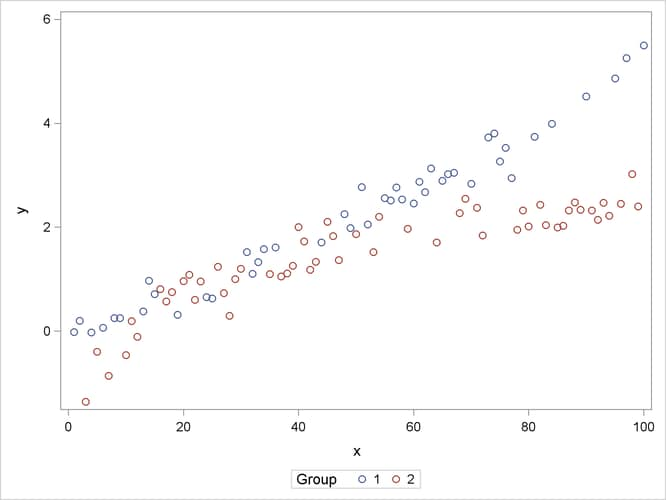The trends in the two groups exhibit curvature, but the type of curvature is not the same in the groups. Also, there appear to be ranges of x values where the groups are similar and areas where the point scatters separate. To model the trends in the two groups separately and with flexibility, you might want to allow for some smooth trends in `x` that vary by `group`. Consider the following PROC GLIMMIX statements:

```proc glimmix data=spline outdesign=x;
class group;
effect spl = spline(x);
model y = group spl*group / s noint;
output out=gmxout pred=p;
run;
```

The EFFECT statement defines a constructed effect named `spl` by expanding the `x` into a spline with seven columns. The `group` main effect creates separate intercepts for the groups, and the interaction of the `group` variable with the spline effect creates separate trends. The NOINT option suppresses the intercept. This is not necessary and is done here only for convenience of interpretation. The OUTPUT statement computes predicted values.

The "Parameter Estimates" table contains the estimates of the group-specific "intercepts," the spline coefficients varied by group, and the residual variance ("Scale," Output 45.15.2).

Output 45.15.2: Parameter Estimates in Two-Group Spline Model

The GLIMMIX Procedure

Parameter Estimates
Effect spl group Estimate Standard
Error
DF t Value Pr > |t|
group   1 9.7027 3.1342 86 3.10 0.0026
group   2 6.3062 2.6299 86 2.40 0.0187
spl*group 1 1 -11.1786 3.7008 86 -3.02 0.0033
spl*group 1 2 -20.1946 3.9765 86 -5.08 <.0001
spl*group 2 1 -9.5327 3.2576 86 -2.93 0.0044
spl*group 2 2 -5.8565 2.7906 86 -2.10 0.0388
spl*group 3 1 -8.9612 3.0718 86 -2.92 0.0045
spl*group 3 2 -5.5567 2.5717 86 -2.16 0.0335
spl*group 4 1 -7.2615 3.2437 86 -2.24 0.0278
spl*group 4 2 -4.3678 2.7247 86 -1.60 0.1126
spl*group 5 1 -6.4462 2.9617 86 -2.18 0.0323
spl*group 5 2 -4.0380 2.4589 86 -1.64 0.1042
spl*group 6 1 -4.6382 3.7095 86 -1.25 0.2146
spl*group 6 2 -4.3029 3.0479 86 -1.41 0.1616
spl*group 7 1 0 . . . .
spl*group 7 2 0 . . . .
Scale     0.07352 0.01121 . . .

Because the B-spline coefficients for an observation sum to 1 and the model contains group-specific constants, the last spline coefficient in each group is zero. In other words, you can achieve exactly the same fit with the MODEL statement

```model y = spl*group / noint;
```

or

```model y = spl*group;
```

The following statements graph the observed and fitted values in the two groups (Output 45.15.3):

```proc sgplot data=gmxout;
series  y=p x=x / group=group name="fit";
scatter y=y x=x / group=group;
keylegend "fit" / title="Group";
run;
```

Output 45.15.3: Observed and Predicted Values by Group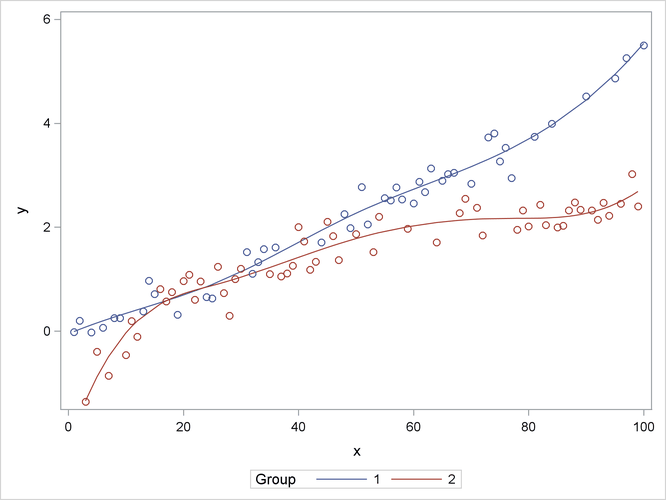Suppose that you are interested in estimating the mean response at particular values of `x` and in performing comparisons of predicted values. The following program uses ESTIMATE statements with nonpositional syntax to accomplish this:

```proc glimmix data=spline;
class group;
effect spl = spline(x);
model y = group spl*group / s noint;
estimate 'Group 1, x=20' group 1    group*spl [1,1 20] / e;
estimate 'Group 2, x=20' group 0  1 group*spl [1,2 20];
estimate 'Diff at x=20 ' group 1 -1 group*spl [1,1 20] [-1,2 20];
run;
```

The first ESTIMATE statement predicts the mean response at x = 20 in group 1. The E option requests the coefficient vector for this linear combination of the parameter estimates. The coefficient for the `group` effect is entered with positional (standard) syntax. The coefficients for the `group`*`spl` effect are formed based on nonpositional syntax. Because this effect comprises the interaction of a standard effect (`group`) with a constructed effect, the values and levels for the standard effect must precede those for the constructed effect. A similar statement produces the predicted mean at x = 20 in group 2.

The GLIMMIX procedure interprets the syntax

```group*spl [1,2 20]
```

as follows: construct the spline basis at x = 20 as appropriate for group 2; then multiply the resulting coefficients for these columns of thematrix with 1.

The final ESTIMATE statement represents the difference between the predicted values; it is a group comparison at x = 20.

Output 45.15.4: Coefficients from First ESTIMATE Statement

The GLIMMIX Procedure

Coefficients for Estimate
Group 1, x=20
Effect spl group Row1
group   1 1
group   2
spl*group 1 1 0.0021
spl*group 1 2
spl*group 2 1 0.3035
spl*group 2 2
spl*group 3 1 0.619
spl*group 3 2
spl*group 4 1 0.0754
spl*group 4 2
spl*group 5 1
spl*group 5 2
spl*group 6 1
spl*group 6 2
spl*group 7 1
spl*group 7 2

The "Coefficients" table shows how the value 20 supplied in the ESTIMATE statement was expanded into the appropriate spline basis (Output 45.15.4). There is no significant difference between the group means at x = 20 (p = 0.8346, Output 45.15.5).

Output 45.15.5: Results from ESTIMATE Statements

Estimates
Label Estimate Standard
Error
DF t Value Pr > |t|
Group 1, x=20 0.6915 0.09546 86 7.24 <.0001
Group 2, x=20 0.7175 0.07953 86 9.02 <.0001
Diff at x=20 -0.02602 0.1243 86 -0.21 0.8346

The group comparisons you can achieve in this way are comparable to slices of interaction effects with classification effects. There are, however, no preset number of levels at which to perform the comparisons because `x` is continuous. If you add further x values for the comparisons, a multiplicity correction is in order to control the familywise Type I error. The following statements compare the groups at values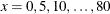and compute simulation-based step-down-adjusted p-values. The results appear in Output 45.15.6. (The numeric results for simulation-based p-value adjustments depend slightly on the value of the random number seed.)

```ods select Estimates;
proc glimmix data=spline;
class group;
effect spl = spline(x);
model y = group spl*group / s;
estimate 'Diff at x= 0' group 1 -1 group*spl [1,1  0] [-1,2  0],
'Diff at x= 5' group 1 -1 group*spl [1,1  5] [-1,2  5],
'Diff at x=10' group 1 -1 group*spl [1,1 10] [-1,2 10],
'Diff at x=15' group 1 -1 group*spl [1,1 15] [-1,2 15],
'Diff at x=20' group 1 -1 group*spl [1,1 20] [-1,2 20],
'Diff at x=25' group 1 -1 group*spl [1,1 25] [-1,2 25],
'Diff at x=30' group 1 -1 group*spl [1,1 30] [-1,2 30],
'Diff at x=35' group 1 -1 group*spl [1,1 35] [-1,2 35],
'Diff at x=40' group 1 -1 group*spl [1,1 40] [-1,2 40],
'Diff at x=45' group 1 -1 group*spl [1,1 45] [-1,2 45],
'Diff at x=50' group 1 -1 group*spl [1,1 50] [-1,2 50],
'Diff at x=55' group 1 -1 group*spl [1,1 55] [-1,2 55],
'Diff at x=60' group 1 -1 group*spl [1,1 60] [-1,2 60],
'Diff at x=65' group 1 -1 group*spl [1,1 65] [-1,2 65],
'Diff at x=70' group 1 -1 group*spl [1,1 70] [-1,2 70],
'Diff at x=75' group 1 -1 group*spl [1,1 75] [-1,2 75],
'Diff at x=80' group 1 -1 group*spl [1,1 80] [-1,2 80] /
run;
```

Output 45.15.6: Estimates with Multiplicity Adjustments

The GLIMMIX Procedure

Estimates
Adjustment for Multiplicity: Holm-Simulated
Label Estimate Standard
Error
DF t Value Pr > |t| Adj P
Diff at x= 0 12.4124 4.2130 86 2.95 0.0041 0.0210
Diff at x= 5 1.0376 0.1759 86 5.90 <.0001 <.0001
Diff at x=10 0.3778 0.1540 86 2.45 0.0162 0.0554
Diff at x=15 0.05822 0.1481 86 0.39 0.6952 0.9043
Diff at x=20 -0.02602 0.1243 86 -0.21 0.8346 0.9578
Diff at x=25 0.02014 0.1312 86 0.15 0.8783 0.9578
Diff at x=30 0.1023 0.1378 86 0.74 0.4600 0.7419
Diff at x=35 0.1924 0.1236 86 1.56 0.1231 0.2890
Diff at x=40 0.2883 0.1114 86 2.59 0.0113 0.0465
Diff at x=45 0.3877 0.1195 86 3.24 0.0017 0.0098
Diff at x=50 0.4885 0.1308 86 3.74 0.0003 0.0022
Diff at x=55 0.5903 0.1231 86 4.79 <.0001 <.0001
Diff at x=60 0.7031 0.1125 86 6.25 <.0001 <.0001
Diff at x=65 0.8401 0.1203 86 6.99 <.0001 <.0001
Diff at x=70 1.0147 0.1348 86 7.52 <.0001 <.0001
Diff at x=75 1.2400 0.1326 86 9.35 <.0001 <.0001
Diff at x=80 1.5237 0.1281 86 11.89 <.0001 <.0001

There are significant differences at the low end and high end of the x range. Notice that without the multiplicity adjustment you would have concluded at the 0.05 level that the groups are significantly different at x = 10. At the 0.05 level, the groups separate significantly for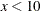and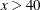.